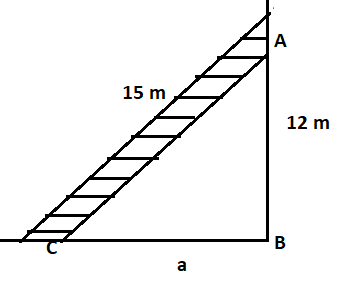Courses
Courses for Kids
Free study material
Offline Centres
MoreLast updated date: 27th Nov 2023
Total views: 379.5k
Views today: 9.79k

# A 15m long ladder reached a window 12m high from the ground on placing it against a wall at a distance a. Find the distance of the foot of the ladder from the wall.Verified
379.5k+ views
Hint: Wall and ground are always perpendicular to each other. So, let us apply Pythagoras theorem to find the value of a.

Now as referenced from the given figure that,
The distance of the window from the ground is 12 m (AB = 12 m).
And, the length of the ladder is 15 m (AC = 15 m).
Now as we know that walls and ground are always perpendicular to each other.
So, ABC triangle formed would be a right-angled triangle, right-angled at B and the hypotenuse of the triangle ABC will be the side opposite to the right angle (i.e. AC).
So, we can apply the Pythagorean theorem in the triangle ABC.
According to Pythagoras theorem, if XYZ is any right-angled triangle, right-angled at Y. And XZ is the hypotenuse of triangle XYZ.
Then, ${\left( {{\text{XZ}}} \right)^{\text{2}}}{\text{ = }}{\left( {{\text{XY}}} \right)^{\text{2}}}{\text{ + }}{\left( {{\text{YZ}}} \right)^{\text{2}}}$
So, applying Pythagoras theorem in triangle ABC. We get,
${\left( {{\text{AC}}} \right)^{\text{2}}}{\text{ = }}{\left( {{\text{AB}}} \right)^{\text{2}}}{\text{ + }}{\left( {{\text{BC}}} \right)^{\text{2}}}$ (1)
Now we had to find the value of BC using equation 1.
${\left( {{\text{BC}}} \right)^{\text{2}}}{\text{ = }}{\left( {{\text{AC}}} \right)^{\text{2}}}{\text{ - }}{\left( {{\text{AB}}} \right)^{\text{2}}}$
Now putting the value of AC and AB in the above equation. We get,
${\left( {{\text{BC}}} \right)^{\text{2}}}{\text{ = }}{\left( {15} \right)^{\text{2}}}{\text{ - }}{\left( {12} \right)^{\text{2}}}{\text{ }} = {\text{ }}225{\text{ }} - {\text{ }}144{\text{ }} = {\text{ }}81$
Now, taking the square root of both sides of the above equation. We get,
BC = 9 m (Because distance cannot be negative)
Hence, the distance of the foot of the ladder from the wall is 9m.

Note: Whenever we come up with a type of problem then first, we check whether there was any angle given between two lines (like ladder or walls). Then after that if the angle is ${90^0}$ then the best way to find the distance is by using the Pythagorean theorem in a right-angled triangle.